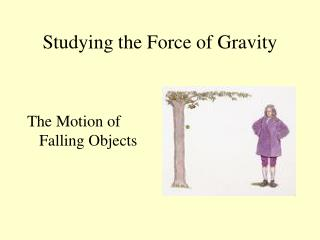# Studying the Force of Gravity - PowerPoint PPT PresentationDownload PresentationStudying the Force of Gravity

Studying the Force of GravityDownload Presentation## Studying the Force of Gravity

- - - - - - - - - - - - - - - - - - - - - - - - - - - E N D - - - - - - - - - - - - - - - - - - - - - - - - - - -
##### Presentation Transcript

1. Studying the Force of Gravity The Motion of Falling Objects

2. Every object exerts a force on every other object. This force is called….. Gravity

3. The Effects of Gravity on Matter • Gravity: a force of attraction between objects that is due to their masses.

4. Chapter 5 Sir Isaac Newton and the Study of Gravity • Observation: an apple falling from a tree • Conclusion 1: an unbalanced force on the apple made the apple fall • Conclusion 2: an unbalanced force on the moon keeps the moon moving around the Earth. • 1665: proposed that these two forces are actually the same force––gravity.

5. Newton and the Study of Gravity • Newton summarized his ideas into the Law of Universal Gravitation. This law describes the relationships between • gravitational force • mass • distance • It is called universal because it applies to all objects in the universe.

6. The Mass of the Objects

7. Distance between the objects

8. Projectile Motion Curved path an object follows when it is thrown or propelled near the surface of the earth

9. Examples • A frog leaping • A swimmer diving • Balls being juggled • Water sprayed by a sprinkler • An arrow shot by an archer

10. Horizontal Component • parallel to ground • Velocity is constant

11. Vertical Component • Acts perpendicular to the ground • Gravity pulls at acceleration of 9.8m/sec2 • Downward acceleration of a thrown object and a falling object are the same

12. Center of Gravity

13. Its weight seems to be concentrated at one point. This point is called…. Center of Gravity Regardless of the size and shape of an object………

14. It is as if….. The force of attraction between the Earth and the object were acting at this one point alone.

15. Galileo • 1564 – 1642 • Most famous for invention of the telescope. • Discovered the moons of Jupiter and the rings of Saturn

16. Galileo’s work on the motion of objects and their acceleration due to gravity paved the way for Isaac Newton’s theories.

17. Galileo proved his theory by rolling balls of different masses down an inclined plane.

18. Galileo’s Experiments proved that gravity causes objects to… Accelerate

19. Chapter 5 Acceleration • Acceleration: the rate at which velocity changes over time • an object accelerates if its speed, or direction, or both change. • positive acceleration: an increase in velocity • negative acceleration, or deceleration: decrease in velocity

20. On Earth, falling objects accelerate at a rate of….. 9.8 m/s2 Galileo was able to determine the correct mathematical law for acceleration due to gravity.

21. This means each second it falls, it will be traveling 9.8 meters per second faster

22. If something is dropped from The Empire State Building (443.00 meters up) it will hit the ground in 9.51 seconds. • It will be traveling at 93.18 meters per second when it hits the ground. • 93.18 meters per second is 208.44 miles per hour (mph).

23. So… the speed of a falling object depends on…. How Long it Falls!

24. To determine acceleration due to gravity… Accleration = Velocity(F) - Velocity(I) Time

25. Velocity: Direction Matters • Velocity: speed of an object in a particular direction • An object’s velocity is constant only if its speed and direction don’t change.

26. Time is on the horizontal axis, and speed is on the vertical axis. Interpreting Graphs: What variable is on the horizontal axis? The vertical axis? Free Fall

27. The slope is 9.8. The speed increases by 9.8 m/s each second. Calculating: Calculate the slope of the graph. What does the slope tell you about the object’s motion? Free Fall

28. 58.8 m/s Predicting: What will the speed of the object be at 6 seconds? Free Fall

29. The speed values would not change. Drawing Conclusions: Suppose another object of the same size but with a greater mass was dropped instead. How would the speed values change? Free Fall

30. Let’s compare and contrast friction and gravity by completing this table Friction Gravity Pulls objects toward one another Opposes motion Effect on motion Types of surfaces involved, how hard the surfaces push together Depends on Mass and distance Measured in Newtons Newtons

31. On Earth, falling objects are affected by air resistance. This opposes downward motion.

32. - Friction and Gravity Air Resistance • Falling objects with a greater surface area experience more air resistance.

33. Increases And the speed of the falling body.. Increases at a slower rate. As a body falls, air resistance..

34. A point is reached where acceleration stops. The highest speed reached by a falling object is called…. Terminal Velocity

35. The object continues to fall at a constant speed.

36. Air resistance increases asthe speed increases. Eventually, the force R ofair resistance becomes equal to the force exertedby the earth, and theobject reaches equilibrium

37. At Terminal VelocityNet Force = 0